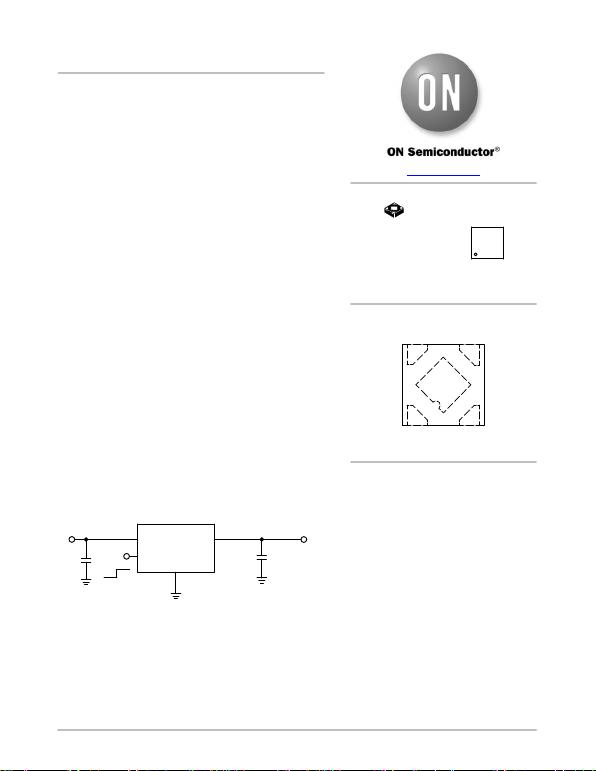© Semiconductor Components Industries, LLC, 2015
April, 2017 − Rev. 9 1Publication Order Number:
NCP707/D
NCP707
200 mA, Very-Low
Quiescent Current, IQ 25 mA,
Low Noise, Low Dropout
Regulator
The NCP707 is 200 mA LDO that provides the engineer with a very
stable, accurate voltage with very low noise suitable for space
constrained, noise sensitive applications. In order to optimize
performance for battery operated portable applications, the NCP707
employs the dynamic quiescent current adjustment for very low IQ
Features
Operating Input Voltage Range: 1.8 V to 5.5 V
Available in Fixed Voltage Options: 1.5 V to 3.3 V
Contact Factory for Other Voltage Options
Very Low Quiescent Current of Typ. 25 mA
Very Low Noise: 22 mVRMS from 100 Hz to 100 kHz
Very Low Dropout: 100 mV Typical at 200 mA
High Power Supply Ripple Rejection: 70 dB at 1 kHz
Thermal Shutdown and Current Limit Protections
Stable with a 1 mF Ceramic Output Capacitor
Available in XDFN 1.0 x 1.0 mm Package
These Devices are Pb−Free, Halogen Free/BFR Free and are RoHS
Compliant
Typical Applicaitons
PDAs, Mobile phones, GPS, Smartphones
Wireless Handsets, Wireless LAN, Bluetooth®, Zigbee®
Portable Medical Equipment
Other Battery Powered Applications
Figure 1. Typical Application Schematic
NCP707
IN
EN
OUT
GND
OFF ON
VOUT
COUT
1 mF
Ceramic
CIN
VIN
XDFN4
MX SUFFIX
CASE 711AJ
MARKING
DIAGRAM
www.onsemi.com
See detailed ordering and shipping information in the package
dimensions section on page 18 of this data sheet.
ORDERING INFORMATION
PIN CONNECTIONS
1
XX = Specific Device Code
M = Date Code
XX M
1
43
21
OUT GND
IN EN
(Top View)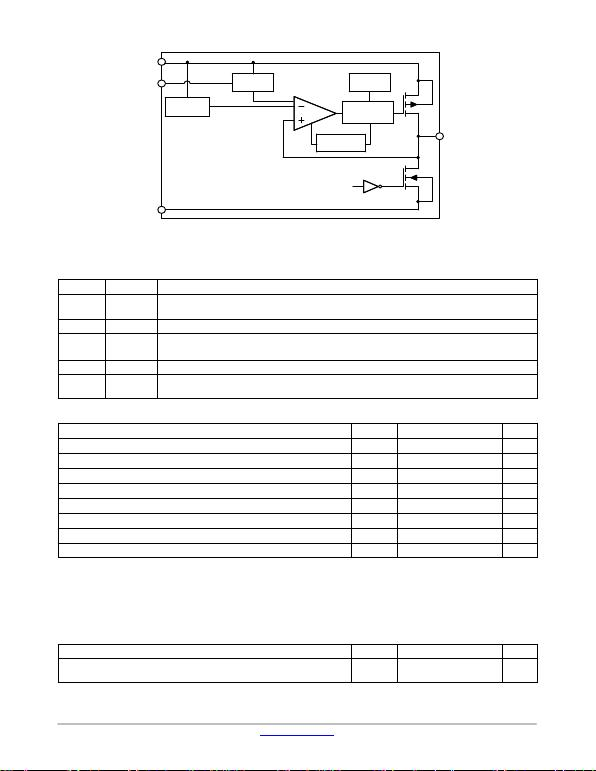NCP707
www.onsemi.com
2
IN
OUT
VOLTAGE
REFERENCE
ACTIVE
DISCHARGE*
MOSFET
DRIVER WITH
CURRENT LIMIT
THERMAL
SHUTDOWN
ENABLE
LOGIC
GND
AUTO LOW
POWER MODE
EN
EN
Figure 2. Simplified Schematic Block Diagram
*Active output discharge function is present only in NCP707AMXyyyTCG and NCP707CMXyyyTCG devices.
yyy denotes the particular VOUT option.
PIN FUNCTION DESCRIPTION
Pin No. Pin Name Description
1 OUT Regulated output voltage pin. A small ceramic capacitor with minimum value of 1 mF is needed from this
pin to ground to assure stability.
2 GND Power supply ground.
3 EN Driving EN over 0.9 V turns on the regulator. Driving EN below 0.4 V puts the regulator into shutdown
mode.
4 IN Input pin. A small 1 mF capacitor is needed from this pin to ground to assure stability.
EPAD Exposed pad should be connected directly to the GND pin. Soldered to a large ground copper plane allows
for effective heat removal.
ABSOLUTE MAXIMUM RATINGS
Rating Symbol Value Unit
Input Voltage (Note 1) VIN −0.3 V to 6 V V
Output Voltage VOUT −0.3 V to VIN + 0.3 V V
Enable Input VEN −0.3 V to VIN + 0.3 V V
Output Short Circuit Duration tSC s
Maximum Junction Temperature TJ(MAX) 150 °C
Storage Temperature TSTG −55 to 150 °C
ESD Capability, Human Body Model (Note 2) ESDHBM 2000 V
ESD Capability, Machine Model (Note 2) ESDMM 200 V
Stresses exceeding those listed in the Maximum Ratings table may damage the device. If any of these limits are exceeded, device functionality
should not be assumed, damage may occur and reliability may be af fected.
1. Refer to ELECTRICAL CHARACTERISTIS and APPLICATION INFORMATION for Safe Operating Area.
2. This device series incorporates ESD protection and is tested by the following methods:
ESD Human Body Model tested per EIA/JESD22−A114
ESD Machine Model tested per EIA/JESD22−A115
Latchup Current Rating tested per JEDEC standard: JESD78
THERMAL CHARACTERISTICS
Rating Symbol Value Unit
Thermal Characteristics, XDFN4 1x1 mm
Thermal Resistance, Junction−to−Air RqJA 250 °C/W
3. Single component mounted on 2 oz, FR4 PCB with 100 mm2 Cu area.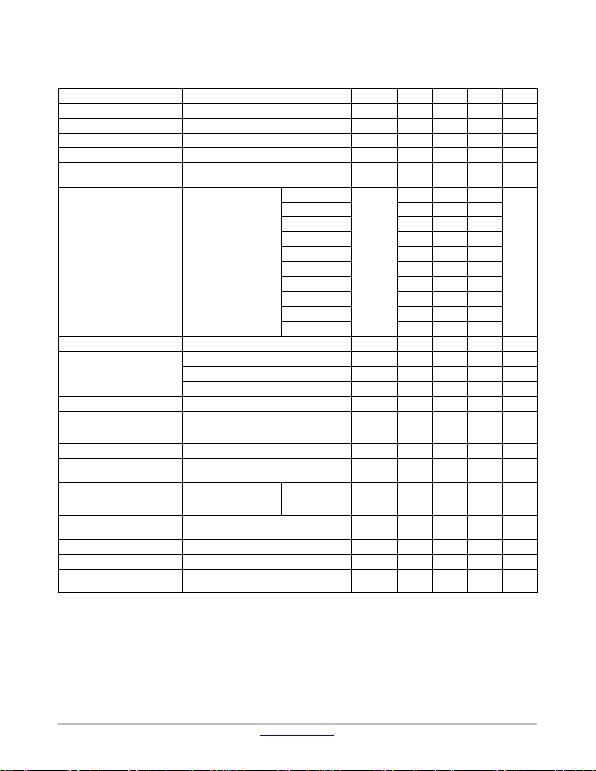NCP707
www.onsemi.com
3
ELECTRICAL CHARACTERISTICS
−40°C TJ 125°C; VIN = VOUT(NOM) + 0.5 V or 1.9 V, whichever is greater; IOUT = 10 mA, CIN = COUT = 1 mF, unless otherwise noted.
VEN = 0.9 V. Typical values are at TJ = +25°C. Min./Max. are for TJ = −40°C and TJ = +125°C respectively (Note 4).
Parameter Test Conditions Symbol Min Typ Max Unit
Operating Input Voltage VIN 1.8 5.5 V
Output Voltage Accuracy VOUT + 0.5 V VIN 5.5 V, IOUT = 0 − 200 mA VOUT −2 +2 %
Line Regulation VOUT + 0.5 V VIN 5.5 V, IOUT = 10 mA RegLINE 400 mV/V
Load Regulation IOUT = 0 mA to 200 mA RegLOAD 10 mV/mA
Load Transient IOUT = 1 mA to 200 mA or 200 mA to 1 mA in
1 ms, COUT = 1 mFTranLOAD 75 mV
Dropout Voltage (Note 5) IOUT = 200 mA
VOUT = 1.5 V
VDO
415 490
mV
VOUT = 1.8 V 221 380
VOUT = 1.85 V 218 370
VOUT = 2.5 V 135 225
VOUT = 2.8 V 118 175
VOUT = 2.85 V 114 170
VOUT = 3.0 V 111 165
VOUT = 3.1 V 107 160
VOUT = 3.2 V 105 155
VOUT = 3.3 V 100 150
Output Current Limit VOUT = 90% VOUT(nom) ICL 250 379 500 mA
Ground Current
IOUT = 0 mA IQ25 35 mA
IOUT = 2 mA IGND 105 mA
IOUT = 200 mA IGND 240 mA
Shutdown Current VEN 0.4 V, VIN = 5.5 V IDIS 0.01 1 mA
EN Pin Threshold Voltage
High Threshold
Low Threshold VEN Voltage increasing
VEN Voltage decreasing VEN_HI
VEN_LO 0.9 0.4
V
EN Pin Input Current VEN = 5.5 V IEN 180 500 nA
Turn−on Time COUT = 1.0 mF, From assertion of VEN to 98%
VOUT(NOM) tON 200 ms
Power Supply Rejection Ratio VIN = 3.6 V, VOUT = 3.1 V
IOUT = 150 mA f = 100 Hz
f = 1 kHz
f = 10 kHz
PSRR 58
70
55
dB
Output Noise Voltage VOUT = 3.1 V, VIN = 3.6 V, IOUT = 200 mA
f = 100 Hz to 100 kHz VN22 mVrms
Thermal Shutdown Temperature Temperature increasing from TJ = +25°C TSD 160 °C
Thermal Shutdown Hysteresis Temperature falling from TSD TSDH 20 °C
Active Output Discharge Resist-
ance VEN < 0.4 V, Version A only
VEN < 0.4 V, Version C only RDIS 1.2
120 kW
W
Product parametric performance is indicated in the Electrical Characteristics for the listed test conditions, unless otherwise noted. Product
performance may not be indicated by the Electrical Characteristics if operated under different conditions.
4. Performance guaranteed over the indicated operating temperature range by design and/or characterization. Production tested at
TJ = T A = 25°C. Low duty cycle pulse techniques are used during testing to maintain the junction temperature as close to ambient as possible.
5. Characterized when VOUT falls 100 mV below the regulated voltage at VIN = VOUT(NOM) + 0.5 V.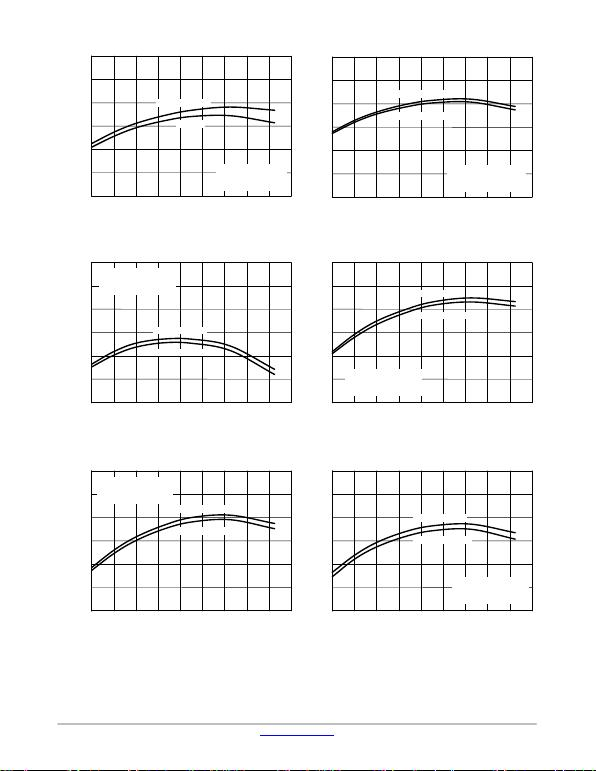NCP707
www.onsemi.com
4
Figure 3. Output Voltage vs. Temperature
VOUT = 1.5 V Figure 4. Output Voltage vs. Temperature
VOUT = 1.85 V
Figure 5. Output Voltage vs. Temperature
VOUT = 2.85 V Figure 6. Output Voltage vs. Temperature
VOUT = 3.0 V
Figure 7. Output Voltage vs. Temperature
VOUT = 3.1 V Figure 8. Output Voltage vs. Temperature
VOUT = 3.3 V
1.510
1.505
1.500
1.495
1.490
1.485
1.480
−40 −20 0 14012010020 40 60 80
JUNCTION TEMPERATURE (°C)
OUTPUT VOLTAGE (V)
IOUT = 10 mA
IOUT
CIN = COUT = 1 mF
VIN = 2.0 V
VOUT(NOM) = 1.5 V
JUNCTION TEMPERATURE (°C)
OUTPUT VOLTAGE (V)
1.860
−40 −20 0 14012010020 40 60 80
1.855
1.850
1.845
1.840
1.835
1.830
CIN = COUT = 1 mF
VIN = 2.35 V
VOUT(NOM) = 1.85 V
IOUT = 10 mA
IOUT = 200 mA
2.870
−40 −20 0 14012010020 40 60 80
JUNCTION TEMPERATURE (°C)
OUTPUT VOLTAGE (V)
2.865
2.860
2.855
2.850
2.845
2.840
IOUT = 10 mA
IOUT = 200 mA
CIN = COUT = 1 mF
VIN = 3.35 V
VOUT(NOM) = 2.85 V
JUNCTION TEMPERATURE (°C)
OUTPUT VOLTAGE (V)
3.000
−40 −20 0 14012010020 40 60 80
2.995
2.990
2.985
2.980
2.975
2.970
IOUT = 10 mA
IOUT = 200 mA
CIN = COUT = 1 mF
VIN = 3.5 V
VOUT(NOM) = 3.0 V
3.110
−40 −20 0 14012010020 40 60 80
JUNCTION TEMPERATURE (°C)
OUTPUT VOLTAGE (V)
3.105
3.100
3.095
3.090
3.085
3.080
CIN = COUT = 1 mF
VIN = 3.6 V
VOUT(NOM) = 3.1 V IOUT = 10 mA
IOUT = 200 mA
JUNCTION TEMPERATURE (°C)
OUTPUT VOLTAGE (V)
3.300
−40 −20 0 14012010020 40 60 80
3.295
3.290
3.285
3.280
3.275
3.270
IOUT = 10 mA
IOUT = 200 mA
CIN = COUT = 1 mF
VIN = 3.8 V
VOUT(NOM) = 3.3 V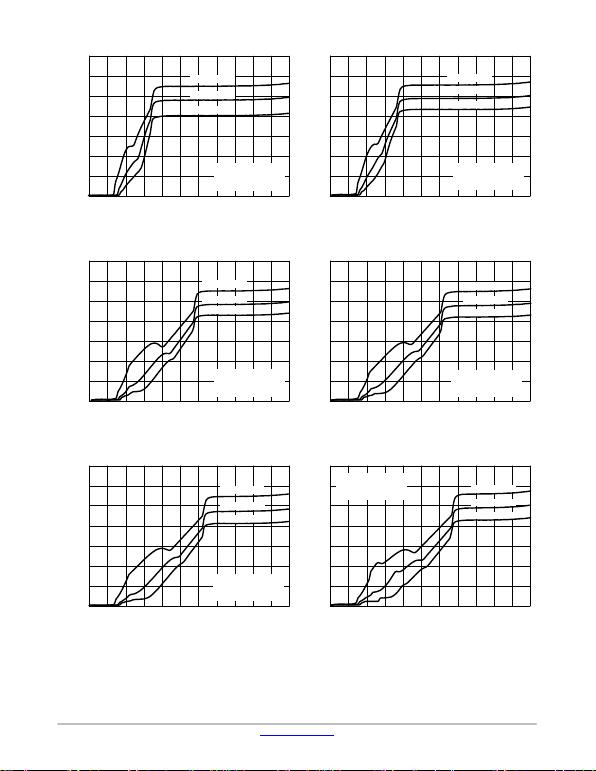NCP707
www.onsemi.com
5
Figure 9. Quiescent Current vs. Input Voltage
VOUT = 1.5 V Figure 10. Quiescent Current vs. Input Voltage
VOUT = 1.8 V
Figure 11. Quiescent Current vs. Input Voltage
VOUT = 2.8 V Figure 12. Quiescent Current vs. Input Voltage
VOUT = 3.0 V
Figure 13. Quiescent Current vs. Input Voltage
VOUT = 3.1 V Figure 14. Quiescent Current vs. Input Voltage
VOUT = 3.3 V
35
0 0.5 1 5.554.51.5 2 2.5 4
INPUT VOLTAGE (V)
QUIESCENT CURRENT (mA)
30
25
20
15
10
5
03.53
TA = 125°C
CIN = COUT = 1 mF
IOUT = 0 mA
VOUT(NOM) = 1.5 V
TA = 25°C
TA = −40°C
35
0 0.5 1 5.554.51.5 2 2.5 4
INPUT VOLTAGE (V)
QUIESCENT CURRENT (mA)
30
25
20
15
10
5
03.53
CIN = COUT = 1 mF
IOUT = 0 mA
VOUT(NOM) = 1.8 V
TA = 125°C
TA = 25°C
TA = −40°C
35
0 0.5 1 5.554.51.5 2 2.5 4
INPUT VOLTAGE (V)
QUIESCENT CURRENT (mA)
30
25
20
15
10
5
03.53
CIN = COUT = 1 mF
IOUT = 0 mA
VOUT(NOM) = 2.8 V
TA = 125°C
TA = 25°C
TA = −40°C
35
0 0.5 1 5.554.51.5 2 2.5 4
INPUT VOLTAGE (V)
QUIESCENT CURRENT (mA)
30
25
20
15
10
5
03.53
TA = 125°C
TA = 25°C
TA = −40°C
CIN = COUT = 1 mF
IOUT = 0 mA
VOUT(NOM) = 3.0 V
35
0 0.5 1 5.554.51.5 2 2.5 4
INPUT VOLTAGE (V)
QUIESCENT CURRENT (mA)
30
25
20
15
10
5
03.53
TA = 125°C
TA = 25°C
TA = −40°C
CIN = COUT = 1 mF
IOUT = 0 mA
VOUT(NOM) = 3.1 V
35
0 0.5 1 5.554.51.5 2 2.5 4
INPUT VOLTAGE (V)
QUIESCENT CURRENT (mA)
30
25
20
15
10
5
03.53
TA = 125°C
TA = 25°C
TA = −40°C
CIN = COUT = 1 mF
IOUT = 0 mA
VOUT(NOM) = 3.3 V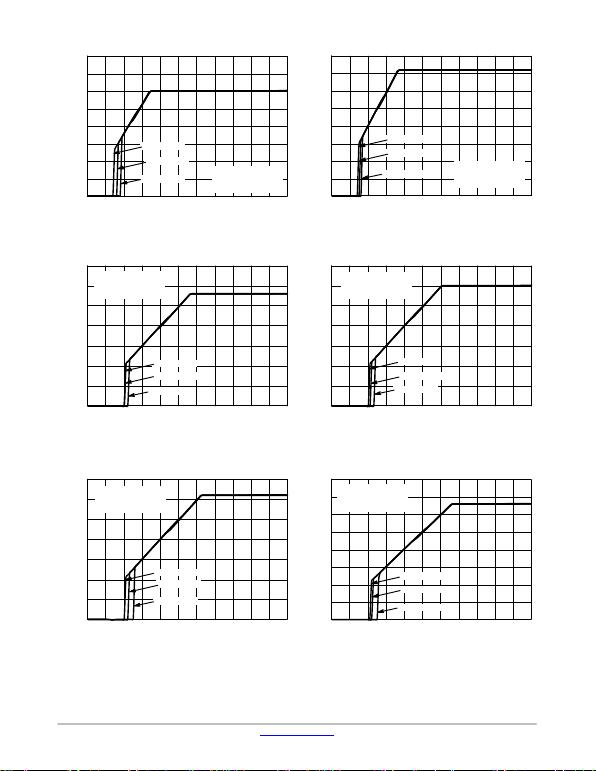NCP707
www.onsemi.com
6
Figure 15. Output Voltage vs. Input Voltage
VOUT = 1.5 V Figure 16. Output Voltage vs. Input Voltage
VOUT = 1.8 V
Figure 17. Output Voltage vs. Input Voltage
VOUT = 2.8 V Figure 18. Output Voltage vs. Input Voltage
VOUT = 3.0 V
Figure 19. Output Voltage vs. Input Voltage
VOUT = 3.1 V Figure 20. Output Voltage vs. Input Voltage
VOUT = 3.3 V
2.00
0 0.5 1 5.554.51.5 2 2.5 4
INPUT VOLTAGE (V)
OUTPUT VOLTAGE (V)
3.53
1.75
1.50
1.25
1.00
0.75
0.50
0.25
0.00
CIN = COUT = 1 mF
IOUT = 0 mA
VOUT(NOM) = 1.5 V
TA = 125°C
TA = 25°C
TA = −40°C
CIN = COUT = 1 mF
IOUT = 0 mA
VOUT(NOM) = 1.8 V
TA = 125°C
TA = 25°C
TA = −40°C
2.00
0 0.5 1 5.554.51.5 2 2.5 4
INPUT VOLTAGE (V)
OUTPUT VOLTAGE (V)
3.53
1.75
1.50
1.25
1.00
0.75
0.50
0.25
0.00
CIN = COUT = 1 mF
IOUT = 0 mA
VOUT(NOM) = 2.8 V
3.50
0 0.5 1 5.554.51.5 2 2.5 4
INPUT VOLTAGE (V)
OUTPUT VOLTAGE (V)
3.53
TA = 125°C
TA = 25°C
TA = −40°C
TA = 125°C
TA = 25°C
TA = −40°C
0 0.5 1 5.554.51.5 2 2.5 43.53
OUTPUT VOLTAGE (V)
3.00
2.50
2.00
1.50
1.00
0.50
0.00
3.50
3.00
2.50
2.00
1.50
1.00
0.50
0.00
CIN = COUT = 1 mF
IOUT = 0 mA
VOUT(NOM) = 3.0 V
INPUT VOLTAGE (V)
3.50
0 0.5 1 5.554.51.5 2 2.5 4
INPUT VOLTAGE (V)
OUTPUT VOLTAGE (V)
3.53
3.00
2.50
2.00
1.50
1.00
0.50
0.00
TA = 125°C
TA = 25°C
TA = −40°C
CIN = COUT = 1 mF
IOUT = 0 mA
VOUT(NOM) = 3.1 V
0 0.5 1 5.554.51.5 2 2.5 43.53
OUTPUT VOLTAGE (V)
4.00
INPUT VOLTAGE (V)
3.50
3.00
2.50
2.00
1.50
1.00
0.50
0.00
CIN = COUT = 1 mF
IOUT = 0 mA
VOUT(NOM) = 3.3 V
TA = 125°C
TA = 25°C
TA = −40°C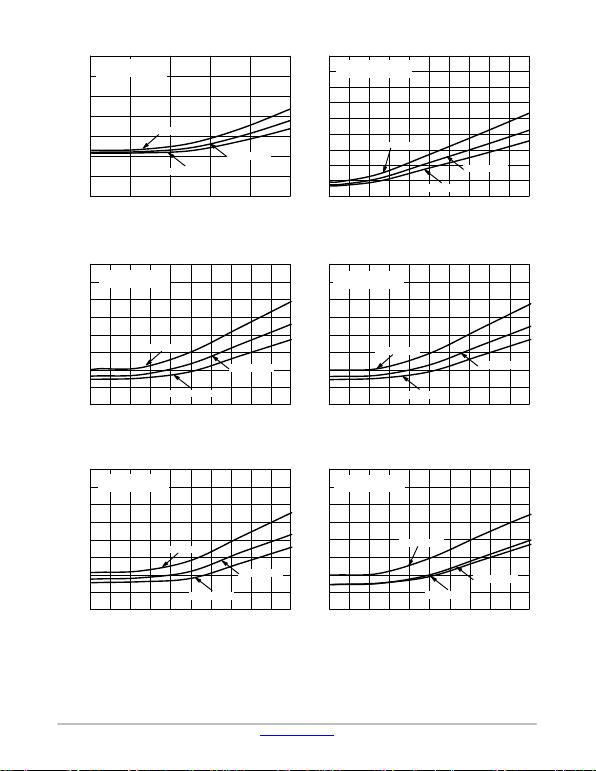NCP707
www.onsemi.com
7
Figure 21. Dropout Voltage vs. Output Current
VOUT = 1.5 V Figure 22. Dropout Voltage vs. Output Current
VOUT = 1.85 V
Figure 23. Dropout Voltage vs. Output Current
VOUT = 2.8 V Figure 24. Dropout Voltage vs. Output Current
VOUT = 3.0 V
Figure 25. Dropout Voltage vs. Output Current
VOUT = 3.1 V Figure 26. Dropout Voltage vs. Output Current
VOUT = 3.3 V
0
DROPOUT VOLTAGE (V)
0.7
OUTPUT CURRENT (A)
0.04 0.20.160.120.08
0.6
0.5
0.4
0.3
0.2
0.1
0
TA = 125°C
CIN = COUT = 1 mF
VOUT(NOM) = 1.5 V
TA = 25°C
TA = −40°C
0
DROPOUT VOLTAGE (V)
0.45
OUTPUT CURRENT (A)
0.04 0.20.160.120.08
0.40
0.35
0.30
0.25
0.20
0.15
0.10
0.05
0
0
DROPOUT VOLTAGE (V)
0.200
OUTPUT CURRENT (A)
0.04 0.20.160.120.08
0.175
0.150
0.125
0.100
0.075
0.050
0.025
0.000
TA = 125°C
TA = 25°C
TA = −40°C
CIN = COUT = 1 mF
VOUT(NOM) = 1.85 V
TA = 125°C
TA = 25°C
TA = −40°C
CIN = COUT = 1 mF
VOUT(NOM) = 2.8 V
0OUTPUT CURRENT (A)
0.04 0.20.160.120.08
0.200
0.175
0.150
0.125
0.100
0.075
0.050
0.025
0.000
DROPOUT VOLTAGE (V)
CIN = COUT = 1 mF
VOUT(NOM) = 3.0 V
TA = 125°C
TA = 25°C
TA = −40°C
0
DROPOUT VOLTAGE (V)
0.200
OUTPUT CURRENT (A)
0.04 0.20.160.120.08
0.175
0.150
0.125
0.100
0.075
0.050
0.025
0.000 0OUTPUT CURRENT (A)
0.04 0.20.160.120.08
0.200
0.175
0.150
0.125
0.100
0.075
0.050
0.025
0.000
DROPOUT VOLTAGE (V)
TA = 125°C
TA = 25°C
TA = −40°C
CIN = COUT = 1 mF
VOUT(NOM) = 3.1 V
TA = 125°C
TA = 25°C
TA = −40°C
CIN = COUT = 1 mF
VOUT(NOM) = 3.3 V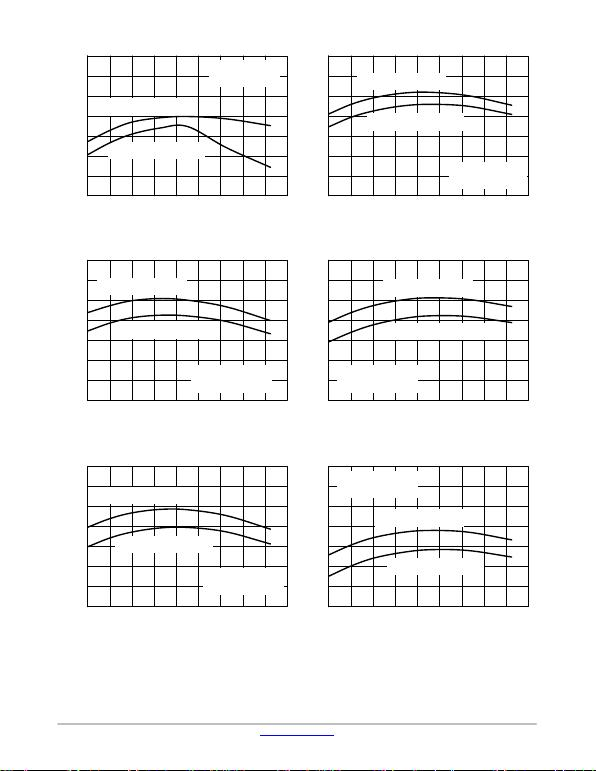NCP707
www.onsemi.com
8
Figure 27. Short−Circuit Limit vs. Temperature
VOUT = 1.5 V Figure 28. Short−Circuit Limit vs. Temperature
VOUT = 1.85 V
Figure 29. Short−Circuit Limit vs. Temperature
VOUT = 2.85 V Figure 30. Short−Circuit Limit vs. Temperature
VOUT = 3.0 V
Figure 31. Short−Circuit Limit vs. Temperature
VOUT = 3.1 V Figure 32. Short−Circuit Limit vs. Temperature
VOUT = 3.3 V
JUNCTION TEMPERATURE (°C)
440
OUTPUT CURRENT (mA)
−40 −20 0 14012010020 40 60 80
420
400
380
360
340
320
300
Short−Circuit Current:
IOUT for VOUT = 0 V
CIN = COUT = 1 mF
VIN = 2.0 V
VOUT(NOM) = 1.5 V
Current Limit: IOUT for
VOUT = VOUT(NOM) − 0.1 V
JUNCTION TEMPERATURE (°C)
440
OUTPUT CURRENT (mA)
−40 −20 0 14012010020 40 60 80
420
400
380
360
340
320
300
Short−Circuit Current:
IOUT for VOUT = 0 V
Current Limit: IOUT for
VOUT = VOUT(NOM) − 0.1 V
CIN = COUT = 1 mF
VIN = 2.35 V
VOUT(NOM) = 1.85 V
JUNCTION TEMPERATURE (°C)
440
OUTPUT CURRENT (mA)
−40 −20 0 14012010020 40 60 80
420
400
380
360
340
320
300
Short−Circuit Current:
IOUT for VOUT = 0 V
CIN = COUT = 1 mF
VIN = 3.35 V
VOUT(NOM) = 2.85 V
Current Limit: IOUT for
VOUT = VOUT(NOM) − 0.1 V
JUNCTION TEMPERATURE (°C)
440
OUTPUT CURRENT (mA)
−40 −20 0 14012010020 40 60 80
420
400
380
360
340
320
300
Short−Circuit Current:
IOUT for VOUT = 0 V
Current Limit: IOUT for
VOUT = VOUT(NOM) − 0.1 V
CIN = COUT = 1 mF
VIN = 3.5 V
VOUT(NOM) = 3.0 V
JUNCTION TEMPERATURE (°C)
440
OUTPUT CURRENT (mA)
−40 −20 0 14012010020 40 60 80
420
400
380
360
340
320
460
Short−Circuit Current:
IOUT for VOUT = 0 V
CIN = COUT = 1 mF
VIN = 3.6 V
VOUT(NOM) = 3.1 V
Current Limit: IOUT for
VOUT = VOUT(NOM) − 0.1 V
JUNCTION TEMPERATURE (°C)
−40 −20 0 14012010020 40 60 80
440
420
400
380
360
340
320
460
OUTPUT CURRENT (mA)
Short−Circuit Current:
IOUT for VOUT = 0 V
Current Limit: IOUT for
VOUT = VOUT(NOM) − 0.1 V
CIN = COUT = 1 mF
VIN = 3.8 V
VOUT(NOM) = 3.3 V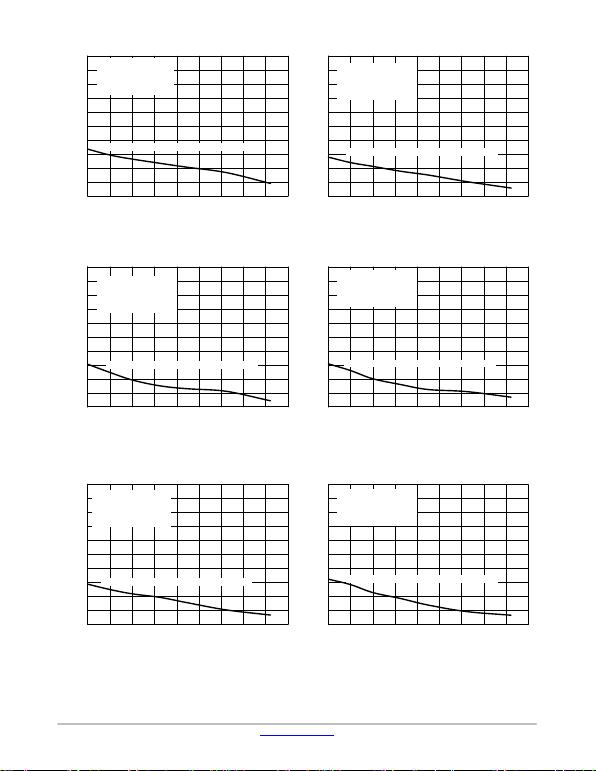NCP707
www.onsemi.com
9
Figure 33. Line Regulation vs. Temperature
VOUT = 1.5 V Figure 34. Line Regulation vs. Temperature
VOUT = 1.85 V
Figure 35. Line Regulation vs. Temperature
VOUT = 2.85 V Figure 36. Line Regulation vs. Temperature
VOUT = 3.0 V
Figure 37. Line Regulation vs. Temperature
VOUT = 3.1 V Figure 38. Line Regulation vs. Temperature
VOUT = 3.3 V
JUNCTION TEMPERATURE (°C)
−40 −20 0 14012010020 40 60 80
5.0
LINE REGULATION (mV)
4.5
4.0
3.5
3.0
2.5
2.0
1.5
1.0
0.5
0.0
Line Regulation from VIN = 2 V to 5.5 V
CIN = COUT = 1 mF
VIN = 2.0 V to 5.5 V
VOUT(NOM) = 1.5 V
IOUT = 10 mA
−40 −20 0 14012010020 40 60 80
JUNCTION TEMPERATURE (°C)
5.0
LINE REGULATION (mV)
4.5
4.0
3.5
3.0
2.5
2.0
1.5
1.0
0.5
0.0
Line Regulation from VIN = 2.35 V to 5.5 V
CIN = COUT = 1 mF
VIN = 2.35 V to 5.5 V
VOUT(NOM) = 1.85 V
IOUT = 10 mA
JUNCTION TEMPERATURE (°C)
−40 −20 0 14012010020 40 60 80
5.0
LINE REGULATION (mV)
4.5
4.0
3.5
3.0
2.5
2.0
1.5
1.0
0.5
0.0
Line Regulation from VIN = 3.35 V to 5.5 V
CIN = COUT = 1 mF
VIN = 3.35 V to 5.5 V
VOUT(NOM) = 2.85 V
IOUT = 10 mA
−40 −20 0 14012010020 40 60 80
JUNCTION TEMPERATURE (°C)
5.0
LINE REGULATION (mV)
4.5
4.0
3.5
3.0
2.5
2.0
1.5
1.0
0.5
0.0
Line Regulation from VIN = 3.5 V to 5.5 V
CIN = COUT = 1 mF
VIN = 3.5 V to 5.5 V
VOUT(NOM) = 3.0 V
IOUT = 10 mA
JUNCTION TEMPERATURE (°C)
−40 −20 0 14012010020 40 60 80
5.0
LINE REGULATION (mV)
4.5
4.0
3.5
3.0
2.5
2.0
1.5
1.0
0.5
0.0
Line Regulation from VIN = 3.6 V to 5.5 V
CIN = COUT = 1 mF
VIN = 3.6 V to 5.5 V
VOUT(NOM) = 3.1 V
IOUT = 10 mA
−40 −20 0 14012010020 40 60 80
JUNCTION TEMPERATURE (°C)
5.0
LINE REGULATION (mV)
4.5
4.0
3.5
3.0
2.5
2.0
1.5
1.0
0.5
0.0
Line Regulation from VIN = 3.8 V to 5.5 V
CIN = COUT = 1 mF
VIN = 3.8 V to 5.5 V
VOUT(NOM) = 3.3 V
IOUT = 10 mA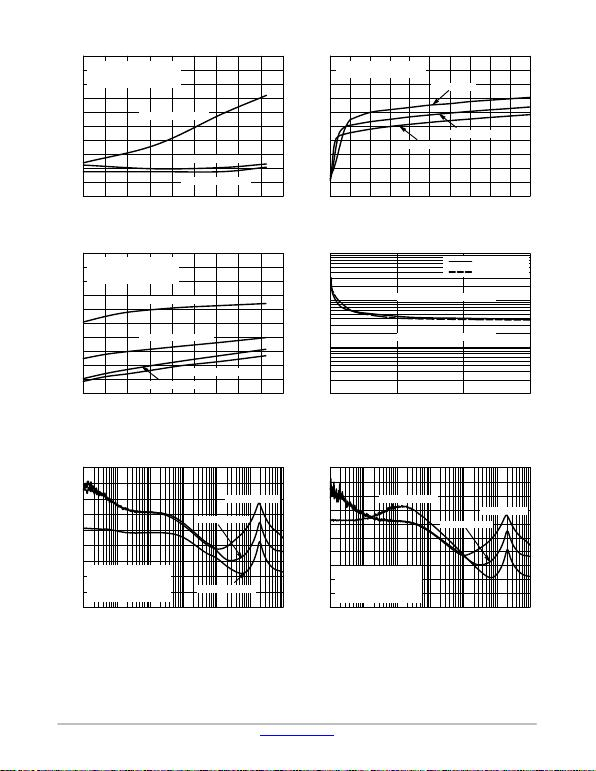NCP707
www.onsemi.com
10
Figure 39. Load Regulation vs. Temperature Figure 40. Ground Current vs. Output Current
Figure 41. Ground Current vs. Temperature Figure 42. Stability vs. Output Capacitor ESR
Figure 43. PSRR vs. Frequency
VOUT = 1.5 V Figure 44. PSRR vs. Frequency
VOUT = 1.85 V
JUNCTION TEMPERATURE (°C)
10
VOUT(NOM) = 1.5 V
CIN = COUT = 1 mF
VIN = VOUT(NOM) + 0.5 V
IOUT = 0 mA to 200 mA
9
8
7
6
5
4
3
2
1
0
−40 −20 0 14012010020 40 60 80
VOUT(NOM) = 1.8 V
VOUT(NOM) = 3.3 V
OUTPUT CURRENT (mA)
200
GROUND CURRENT (mA)
012 10983456
180
160
140
120
100
80
60
40
20
07
TA = 125°C
CIN = COUT = 1 mF
VIN = VOUT(NOM) + 0.5 V
TA = 25°C
TA = −40°C
JUNCTION TEMPERATURE (°C)
300
−40 −20 0 14012010020 40 60 80
GROUND CURRENT (mA)
280
260
240
220
200
CIN = COUT = 1 mF
VIN = VOUT(NOM) + 0.5 V
IOUT = 200 mA
VOUT(NOM) = 1.5 V
VOUT(NOM) = 1.85 V
VOUT(NOM) = 3.3 V
VOUT(NOM) = 2.85 V
OUTPUT CURRENT (mA)
100
CAPACITOR ESR (W)
0
10
1
0.1
0.01 100 200 300
UNSTABLE OPERATION
STABLE OPERATION
VOUT = 1.5 V
VOUT = 3.3 V
FREQUENCY (Hz)
90
10
PSRR (dB)
80
70
60
50
40
30
20
10
0100 1k 10k 100k 1M 10M
COUT = 1 mF
CIN = none,
VIN = 2.0 V ± 50 mVAC
VOUT(NOM) = 1.5 V
IOUT = 1 mA
IOUT = 10 mA
IOUT = 150 mA
FREQUENCY (Hz)
10 100 1k 10k 100k 1M 10M
90
PSRR (dB)
80
70
60
50
40
30
20
10
0
100
IOUT = 150 mA
IOUT = 10 mA
IOUT = 1 mA
COUT = 1 mF
CIN = none,
VIN = 2.35 V ± 50 mVAC
VOUT(NOM) = 1.85 V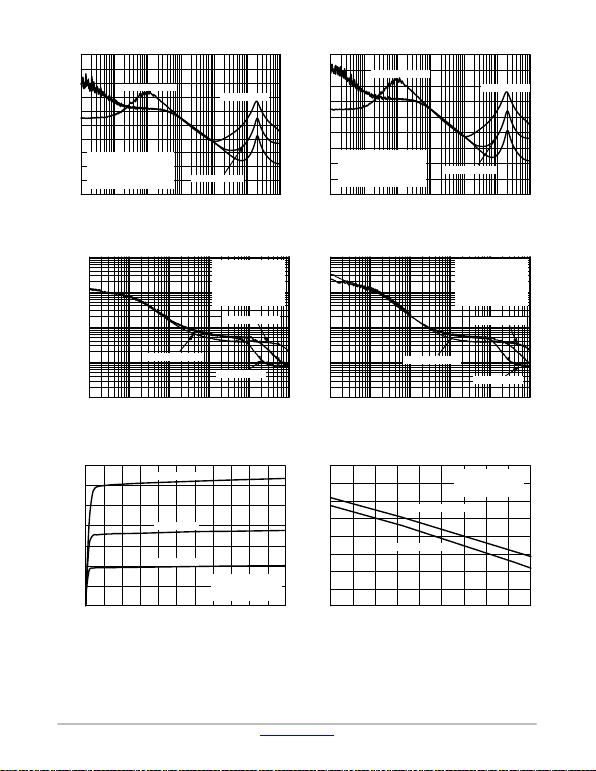NCP707
www.onsemi.com
11
Figure 45. PSRR vs. Frequency
VOUT = 3.0 V Figure 46. PSRR vs. Frequency
VOUT = 3.1 V
Figure 47. Output Noise Density vs. Frequency
VOUT = 1.5 V Figure 48. Output Noise Density vs. Frequency
VOUT = 3.1 V
Figure 49. Enable Input Current vs. Enable
Voltage Figure 50. Enable Threshold Voltage vs.
Temperature
FREQUENCY (Hz)
10 100 1k 10k 100k 1M 10M
90
PSRR (dB)
80
70
60
50
40
30
20
10
0
100
IOUT = 150 mA
IOUT = 10 mA
IOUT = 1 mA
COUT = 1 mF
CIN = none,
VIN = 3.5 V ± 50 mVAC
VOUT(NOM) = 3.0 V
FREQUENCY (Hz)
10 100 1k 10k 100k 1M 10M
90
PSRR (dB)
80
70
60
50
40
30
20
10
0
IOUT = 150 mA
IOUT = 1 mA
IOUT = 10 mA
COUT = 1 mF
CIN = none,
VIN = 3.6 V ± 50 mVAC
VOUT(NOM) = 3.1 V
FREQUENCY (Hz)
10 100 1k 10k 100k 1M
OUTPUT VOLTAGE NOISE (mV/rtHz)
1.000
0.100
0.010
0.001
IOUT = 200 mA
IOUT = 10 mA
IOUT = 1 mA
CIN = COUT = 1 mF
VIN = 2.0 V
VOUT = 1.5 V
MLCC, X7R
1206 size
FREQUENCY (Hz)
10 100 1k 10k 100k 1M
OUTPUT VOLTAGE NOISE (mV/rtHz)
1.000
0.100
0.010
0.001
CIN = COUT = 1 mF
VIN = 3.6 V
VOUT = 3.1 V
MLCC, X7R
1206 size
IOUT = 10 mA
IOUT = 1 mA
IOUT = 200 mA
ENABLE VOLTAGE (V)
0 0.5 1 3.5 4.5 5 5.5
ENABLE CURRENT (mA)
0.35
0.3
0.25
0.2
0.15
0.1
0.05
0431.5 2 2.5
TA = 125°C
TA = 25°C
TA = −40°C
CIN = COUT = 1 mF
VIN = 2 V
VOUT(NOM) = 1.5 V
ENABLE CURRENT (mA)
JUNCTION TEMPERATURE (°C)
−40 −20 100 120 140
0.9
806002040
0.85
0.8
0.75
0.7
0.65
0.6
0.55
0.5
VIN = 2 V
CIN = COUT = 1 mF
VOUT(NOM) = 1.5 V
VEN = Low to High
VEN = High to Low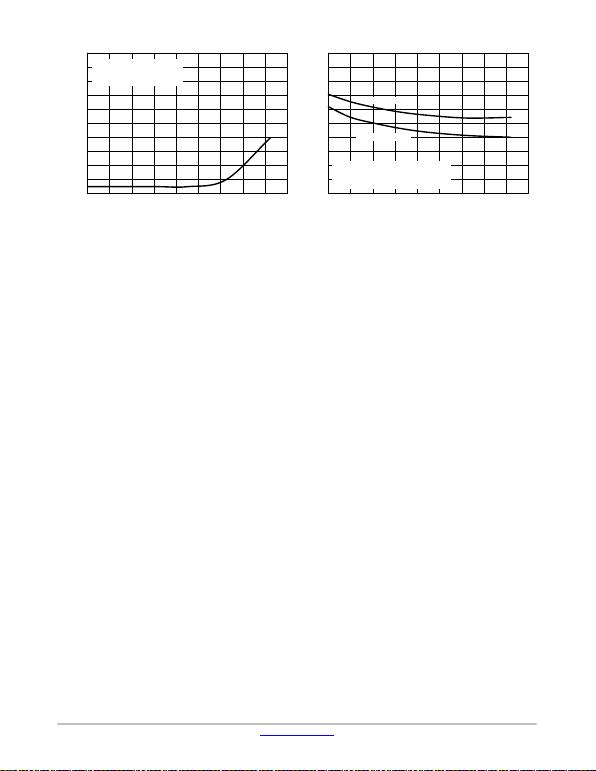NCP707
www.onsemi.com
12
Figure 51. Shutdown Current vs. Temperature Figure 52. VOUT Turn−on Time vs.
Temperature
SHUTDOWN CURRENT (mA)
JUNCTION TEMPERATURE (°C)
−40 −20 100 120 140
0.2
806002040
0.16
0.12
0.08
0.04
0
CIN = COUT = 1 mF
VIN = VOUT(NOM) + 0.5 V
VEN = 0 V
VOUT TURN−ON TIME (ms)
JUNCTION TEMPERATURE (°C)
−40 −20 100 120 140
300
806002040
280
260
240
220
200
180
160
140
120
100
CIN = COUT = 1 mF
VIN = VOUT(NOM) + 0.5 V
VEN = Step from 0 V to 1 V / 1 ms
VOUT = 3.3 V
VOUT = 1.5 V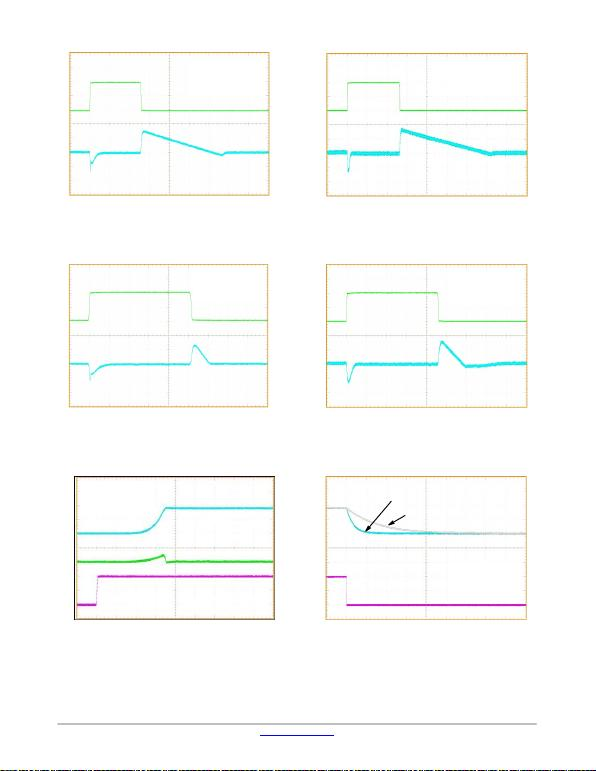NCP707
www.onsemi.com
13
50 mV/div 100 mA / div
30 mV/div 100 mA / div
50 mV/div 100 mA / div
30 mV/div 100 mA / div
100 mA/div 1 V/div
1 V/div
IOUT = 1 mA to 200 mA, COUT = 1 mF
20 ms / div
VOUT
IOUT
VIN = 3.6 V
VOUT(nom) = 3.1 V
CIN = COUT = 1 mF
200 mA
1 mA
IOUT = 1 mA to 200 mA, COUT = 4.7 mF
20 ms / div
VIN = 3.6 V
VOUT(nom) = 3.1 V
CIN = COUT = 4.7 mF
VOUT
IOUT
200 mA
1 mA
IOUT = 10 mA to 200 mA, COUT = 1 mF
10 ms / div
IOUT = 10 mA to 200 mA, COUT = 4.7 mF
20 ms / div
VIN = 3.6 V
VOUT(nom) = 3.1 V
CIN = COUT = 1 mF
VIN = 3.6 V
VOUT(nom) = 3.1 V
CIN = COUT = 4.7 mF
VOUT
IOUT
VOUT
IOUT
200 mA
10 mA
200 mA
10 mA
VOUT = 1.8 V
VIN = 2.3 V
VOUT(nom) = 1.8 V
CIN = COUT = 1 mF
IIN = 1 mA
VEN = 1 V
VOUT = 0 V
VEN = 0 V
IIN
Figure 57. Enable Turn−On Response
VOUT = 1.8 V, COUT = 1 mF
500 ms / div
Figure 58. Enable Turn−Off Response
VOUT = 1.8 V, COUT = 1 mF (A Version)
500 ms / div
VOUT = 0 V
VIN = 2.3 V
VOUT(nom) = 1.8 V
CIN = COUT = 1 mF
VEN = 0 V
VEN = 1 V
VOUT = 1.8 V RL = 1.8 kW
RL = 180 kW